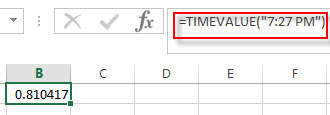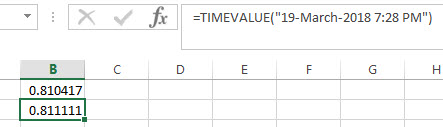# Excel TIMEVALUE Function

This post will guide you how to use Excel TIMEVALUE function with syntax and examples in Microsoft excel.

### Description

The Excel TIMEVALUE function returns the decimal number of the time represented by a text string. so it will convert a time represented by a text string into an Excel time value. and the returned value is range from 0 to 0.99988, it indicated the times from 0:00:00 to 23:59:59.
For example, when you fed the text string “7:13 PM” into TIMEVALUE function, and it returns 0.80069. the returned value is a numeric value and this value can be used to manipulate with another formulas or pivot tables.

The TIMEVALUE function is a build-in function in Microsoft Excel and it is categorized as a DATE and TIME Function.

The TIMEVALUE function is available in Excel 2016, Excel 2013, Excel 2010, Excel 2007, Excel 2003, Excel XP, Excel 2000, Excel 2011 for Mac.

### Syntax

The syntax of the TIMEVALUE function is as below:

`=TIMEVALUE (time_text)`

Where the TIMEVALUE function arguments is:

• Serial_text -This is a required argument. A text string that represents a time, within the text string, the hours, minutes and seconds components should be separated by colon characters.

Note:

• the date information in text string will be ignored.
• if the time_textr is not a valid Excel time in a text format , it will return #VALUE! error.

### Excel TIMEVALUE Function Examples

The below examples will show you how to use Excel TIMEVALUE Function to convert a text string that representing a time in Excel.

1# get decimal part of a day, with only the time portion specified, enter the following formula in Cell B1.

`=TIMEVALUE("7:27 PM")`2# get decimal part of a day, with date and time specified, enter the following formula in Cell B3.

```=TIMEVALUE("19-March-2018 7:28PM")```